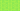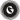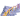PricingResources

# The P/E Ratio ExplainedWritten by

The P/E ratio is an important figure to keep an eye on when it comes to your company’s stocks. By looking at the P/E ratio, you can get a better understanding of the overall value of your business. Read on to have the P/E ratio explained.

## P/E ratio meaning

The P/E ratio meaning is Price Earnings Ratio. It describes the relationship between your stock price as a company – expressed as P (Price) – and your earnings per share (E). You can also look at the P/E ratio of markets. In short, it can be used to show if markets are over or undervaluing stock.

For example, the market price of any given stock will reflect how much people are willing to pay for it, but it doesn’t explain whether this is actual value for money. The P/E ratio, in contrast, tells you whether that stock price is an accurate measure of the company’s value or earnings. However, the P/E ratio may need the added context of your competitors. P/E ratio is used as a research tool when it comes to selecting stock, so to understand if your final figure still seems expensive, you will need to find the P/E ratio of a competitor’s stock as well.

## How to calculate P/E ratio

The P/E ratio can be found using the below P/E ratio formula:

So, let’s say a company has a share price of \$40, and its earnings per share for the past year is \$4, then it has a P/E ratio of 10. For companies that have had losses or no profit, the P/E ratio may not be able to be found, or a negative ratio can be calculated.

Knowing how to calculate P/E ratio also depends on the figure you are looking for. P/E ratio has two types:

• Trailing P/E ratio

• Forward P/E ratio

Trailing P/E ratio is based on previous earnings (over the past 12 months) per share, and can be found using the above formula. It’s also possible to calculate your trailing P/E ratio over longer periods, such as a decade or more.

Forward P/E ratio considers future estimates, the numbers for which are often provided by equity research analysts or found on sites that provide real-time market updates. The forward P/E ratio can, in many cases, be considered the more relevant figure, as buyers are more invested in the earning potential of the stock they are buying into than its past success.

The forward P/E ratio also uses a slightly different formula:

You can also compare the trailing and forward P/E ratio of the same stock to each other. If the forward P/E ratio is higher than trailing, it suggests that analysts expect a decrease in earnings, or vice versa.

## What is a low/high P/E ratio?

As we mentioned, the figures provided by a P/E ratio formula give more meaningful insight if they are compared to other stocks. In general terms, a benchmark can be set using something like the S&P 500 index. A high P/E ratio compared to this average suggests that the company is likely to grow, as is often the case for tech companies. Lower P/E ratios are considered ‘value’ stocks which may not show as much growth.

The P/E ratio is just one of the ways an investor can consider their options, however, and it should not be considered an absolute answer as to where they should place their funds. In some cases, an investor may simply believe that their understanding of a stock’s value is more accurate than the P/E ratio, having considered all the same factors, and take the risk on a low P/E ratio stock anyway.

### We can help

GoCardless helps you automate payment collection, cutting down on the amount of admin your team needs to deal with when chasing invoices. Find out how GoCardless can help you with ad hoc payments or recurring payments.Contact sales

Sales

Support